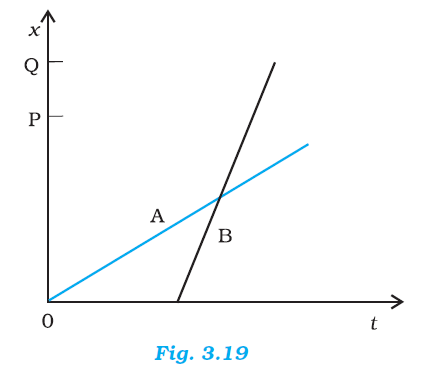## Pages

### Motion in a straight line NCERT Solutions Class 11 Physics - Solved Exercise Question 3.2

Question 3.2

The position-time (x-t) graphs for two children A and B returning from their school O to their homes P and Q respectively are shown in Fig. 3.19. Choose the correct entries in the brackets below:
(a) (A/B) lives closer to the school than (B/A)
(b) (A/B) starts from the school earlier than (B/A)
(c) (A/B) walks faster than (B/A)
(d) A and B reach home at the (same/different) time
(e) (A/B) overtakes (B/A) on the road (once/twice).Solution:

(a) A lives closer to school than B.
(b) A starts from school earlier than B.
(c) B walks faster than A.
(d) A and B reach home at the same time.
(e) B overtakes A once on the road.

Explanation:
(a) In the given xt graph, it can be observed that distance OP < OQ. Hence, the distance of school from the A’s home is less than that from B’s home.

(b) In the given graph, it can be observed that for x = 0, t = 0 for A, whereas for x = 0, t has some finite value for B. Thus, A starts his journey from school earlier than B.

(c) In the given xt graph, it can be observed that the slope of B is greater than that of A. Since the slope of the xt graph gives the speed, a greater slope means that the speed of B is greater than the speed A.

(d) It is clear from the given graph that both A and B reach their respective homes at the same time.

(e) B moves later than A and his/her speed is greater than that of A. From the graph, it is clear that B overtakes A only once on the road.